Courses

# Introduction to Number Series Notes | EduRev

## CAT : Introduction to Number Series Notes | EduRev

The document Introduction to Number Series Notes | EduRev is a part of the CAT Course Logical Reasoning (LR) and Data Interpretation (DI).
All you need of CAT at this link: CAT

Introduction

Number series is a form of sequence, where some numbers are mistakenly put into the series of numbers and some number is missing in that series, we need to observe first and then find the accurate number to that series.

The sequence is the list of numbers written in a specific order.

Example:  1, 4, 9, 16, 25, 36....

Some Common Types and Their TricksType 1 - Addition / Subtraction or Multiplication / Division

Example 1:  19, 23, 39, 75, _, 239

• The most common trick to solve a number series is to solve by checking the difference between two adjacent numbers, but the difference could not only lead to addition/subtraction but it can also be with multiplication/division.
• Therefore, to check whether to think addition wise or multiplication wise in increasing sequence, one must assume with the help of the difference between the first and last number of the given sequence.
• If the difference seems to be less according to the number of steps used to make the last number from first than we should check addition.
• If the difference seems to be large or too large one must check the multiplication trick between the adjacent numbers.

In Example 1, given above, the difference between first no. (19) and last number (239) is 220.

• Now a question will arise how we will assume whether the difference is more or less. We will assume it by keeping in mind the number of steps required to start from the first number till the last number.
• In Example 1, 19 becomes 239 in five steps, because there are four more numbers between them, one of which we have to find out.
• The difference of 220 between 19 and 239 in five steps logically giving priority to addition over multiplication in an increasing sequence like this.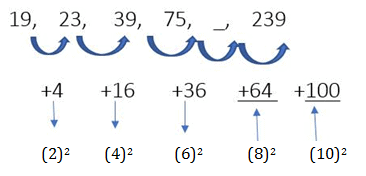• 75 + 64 = 139, 139 + 100 = 239

Question 1:Look at this series: 7, 10, 8, 11, 9, 12, ... What number should come next?

Example 2: 10, 31, 95, 288, ___, 2609
As we can see in the above example, the difference between the first number (10) and the last number (2609) is 2599 in five steps, which indicate us to check multiplication trick between the numbers.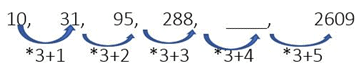(288*3) + 4 = 868, is the correct answer.

Note : While checking multiplication trick always start from right end of the sequence.

Example 3: 30, 34, 43, 59, 84, 120,?
(a) 169
(b) 148
(c) 153
(d) 176
(e) None of these
Ans. (a)
Solution:
The given pattern is:

+4, +9, +16, +25 and so on.
So, missing term is 169 = 120 + 49

Example 4: 40, 54, 82, ?, 180, 250
(a) 142
(b) 124
(c) 136
(d) 163
(e) None of these
Ans. (b)
Solution:
The pattern is: +14, + 28, + 42, + 52, + 70
So, missing term is 82 + 42 = 124

Question 2:Look carefully for the pattern, and then choose which pair of numbers comes next - 8,11, 21, 15, 18, 21, 22

Example 5: 0, 1, 3, 8, 18, 35, 264
(a) 62
(b) 35
(c) 18
(d) 8
(e) None of these
Ans. (a)
Solution: The pattern is +(02+1), +(12+1), + (22+1) ,+ (32+1), + (42+1), + (52+1)
So, 264 is wrong and must be replaced by 35 + (52+1) = 62

Example 6: 1, 9, 125, 49, 729, 121, 2147
(a) 2147
(b) 729
(c) 125
(d) 1
(e) None of these
Ans. (a)

Example 7: 5531, 5506, 5425, 5304, 5135, 4910, 4621
(a) 5531
(b) 5425
(c) 4621
(d) 5135
(e) 5506
Ans. (a)
Solution: The number should be 5555 in place of 5531.
-72, -92, -112, -132, -152, -172…

Example 8: 6, 7, 9, 13, 26, 37, 69
(a) 7
(b) 26
(c) 69
(d) 37
(e) 9
Ans. (b)
Solution: The number should be 21 in place of 26.
The pattern is: +1, +2, +4, +8, +16, +32

Example 9: 1, 3, 10, 36, 152, 760, 4632
(a) 3
(b) 36
(c) 4632
(d) 760
(e) 152
Ans. (d)
Solution: The number should be 770 in place of 760.
The pattern is: ×1 +2, ×2 +4, ×3 +6, ×4 + 8, ×5 +10, ×6 + 12, …

Example 10: 4, 3, 9, 34, 96, 219, 435
(a) 4
(b) 9
(c) 34
(d) 435
(e) 219
Ans. (d)
Solution: The series is 02+ 4, 12+2, 32+0, 62-2, 102-4, 152- 6, 212 – 8…
Hence, 435 should be replaced with 433

Example 11: 157.5, 45, 15, 6, 3, 2, 1
(a) 1
(b) 2
(c) 6
(d) 157.5
(e) 45
Ans. (a)
Solution: The number should be 2 in place of 1.
3.5, 3, 2.5, 2, 1.5, 1

Type 2 - Perfect Square or Perfect Cube

Question: 4, 18, 48, 100, 180, ___.

• In case, if Type-1 is not applicable in a sequence, then in the next step, we must compare given numbers or their differences to square or cube of natural numbers, as in the above example.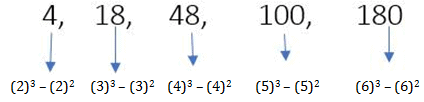• Therefore, (7)3-(7)2 = 294, is the correct answer.

(a) Perfect Square Series: This type of series is based on the square of a number that is in the same order and one square number is missing in that given series.

Question 3:In the following options, a few number series are present. One of them has an error, pick the wrong one out:

Example 1: 841, ?, 2401, 3481, 4761
Answer: 292, 392, 492, 592, 69

Question 4: In the sequence given below, a term is missing. The missing term is written in the options that are present below. Find the missing term and choose it from the options below: 8, 12, 21, 37, __

Example 2: 1, 9, 25, ?, 81, 121
Answer: 12, 32, 52, 72, 92, 112

Example 3: 289, 225, 169, ?, 81
Answer: 172, 152, 132, 112, 92

(b) Perfect Cube Series: This type of series is based on the cube of a number that is in the same order and one cube number is missing in that given series.
Example 1: 3375, ?, 24389, 46656, 79507
Answer: 153, 223, 293, 363, 433
(Each cube digit added with seven to become next cube number)

Example 2: 729, 6859, 24389, ?, 117649, 205379
Answer: 93, 193, 293, 393, 493, 593

Example 3: 1000, 8000, 27000, 64000, ?
Answer: 103, 203, 303, 403, 50

Question 5:In the following series, a number is such that it does not belong to the arrangement.
8000, 27000, 64000, 105000
Select the option that has this number:

Type 3 - Factorisation / Prime Factorisation

• If Type 1 and Type 2 is not applicable in a sequence, one must try to make factors of the numbers in the next step.

Example: 6, 15, 35, 77, 143, __.
In the above example, all the previous tricks are not applicable to get an answer. Hence, We will make factors of the given numbers.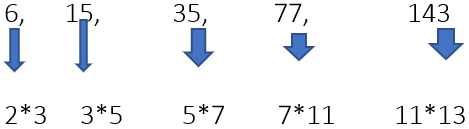(2,3,5,7,11,13) all are prime numbers in ascending order.
Hence, 13*17 = 221, is the correct answer.

Question 6:Next number in series 31, 41, 47, 59, __?

Type 4 - Fibonacci Series

• A series in which a number is made by using previous two numbers are called Fibonacci series.

Example: 1, 4, 5, 9, 14, 23, ___
In the above sequence, all the numbers are the sum of the previous two numbers.
Therefore, 23+14 = 37, is the correct answer.

Type 5 - Sum of Digits

Example 1: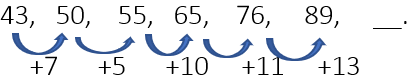In the above sequence, the difference between two numbers is the sum of the digits of the first number.
Hence, 89+17 = 106, is the correct answer.

Example 2: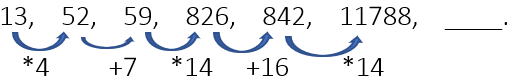All the numbers are multiplied by their sum or added by their sum alternately.
Therefore, 11788 + 25 = 11813, is the correct answer.

Type 6 - Alternate Pattern Series

• When numbers given as a hint in a question are more or when a question asks two numbers of a series or the same number come twice in a series, these all give a hint to alternate pattern series.

Example 1: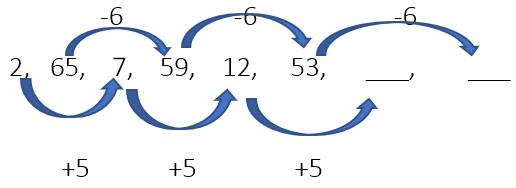So, numbers are 12+5 = 17, 53-6 = 47
Ans. 17, 47

Example 2: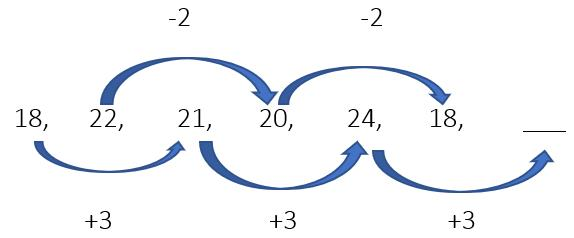So, the number is 24+3 = 27
Ans. 27

Question 7:In the given series, a number is missing. The same number is present in the options below.
32, 40, 24, 16, 24, __
Select the missing number.

Type 7 - Decimal Pattern Series

When the numbers of the sequence are given in the decimal form is decimal pattern series.
Example 1: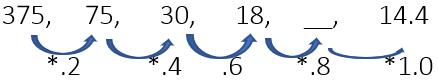So, the answer is 18 * 0.8 = 14.4

Example 2:  16, 24, 60, 210, 945, __
The pattern is:
16 * 1.5 = 24
24 * 2.5 =60
60 * 3.5 = 210
210 * 4.5 = 945
945 * 5.5 = 5197.5

Type 8 - Bracket Pattern Series

While using bracket pattern we multiply first outside and either add or sub based on given number.

Example 1: 3, 28, 180,  ____,  3676
The pattern is :
(3+1)*7 = 28
(28+2)*6 =180
(180+3)*5 = 915
(915+4)*4 = 3676
Ans. 915

Example 2: 37, 31, 52, 144, __, 2810
The pattern is :
(37-6)*1=31
(31-5)*2=52
(52-4)*3=144
(144-3)*4=564
(564-2)*5=2810
Ans. 564

Type 9 - Dual Pattern Series

Example:
15,   9,    8,     12,       36,       170
19,   a,    b,     __,       d,           e,
The pattern is :
(15-6)*1=9
(9-5)*2=8
(8-4)*3=12
Similarly:
(19-6)*1=13
(13-5)*2=16
(16-4)*3=36
Ans. 36

Type 10 - Factorial Based Series

This is the latest pattern question asked in latest exams.

Example 1: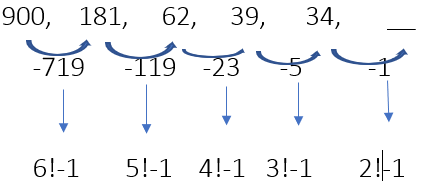Ans. 33

Example 2: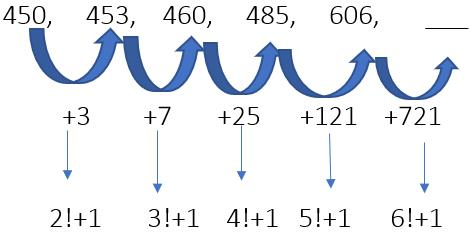Ans. 606 + 721 = 1327

Question 8:​In the given series, only one number is wrong.
3, 9, 19, 32
Find out the wrong number.

Offer running on EduRev: Apply code STAYHOME200 to get INR 200 off on our premium plan EduRev Infinity!

## Logical Reasoning (LR) and Data Interpretation (DI)

48 videos|54 docs|113 tests

,

,

,

,

,

,

,

,

,

,

,

,

,

,

,

,

,

,

,

,

,

;